Basic Practice - Dilations Terms of Use    Contact Person: Donna Roberts1.Is this an example of a dilation?

 yes no

2.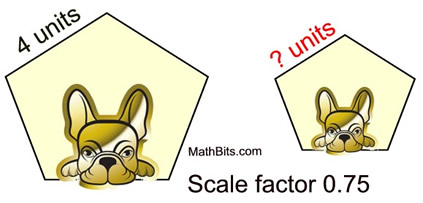Under the dilation shown at the left, the value of the "?" is:
 2 2.5 3

3.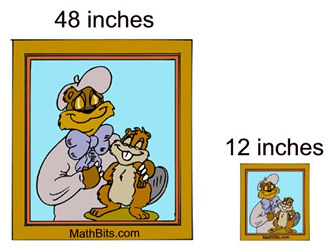Under dilations, the picture shown at the left has been reduced in size. The scalar factor is:
 ¼ ½ 2 4

4.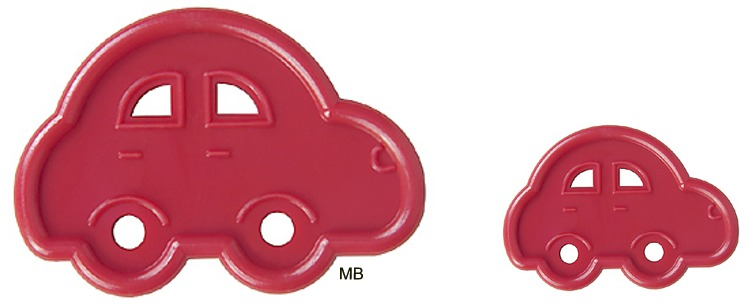The length of the small car is 4 inches. Under a dilation of scale factor of 2 the length of the larger car is:
 2 inches 8 inches

5.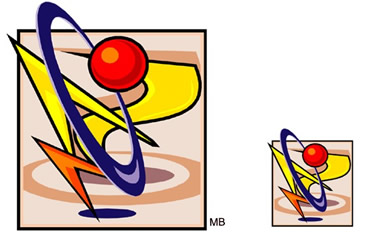The large design shown at the left is 6 feet wide. After a dilation, the design is 2.4 feet wide. The scalar factor for this dilation is:
 3 2.5 0.5 0.4

6.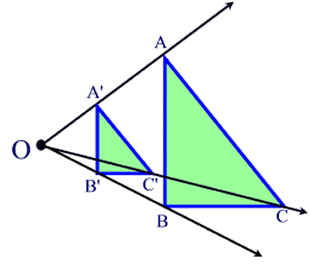ΔA'B'C' is the image of ΔABC under a dilation centered at O of ratio 1/2. Which of the following relationships is true?
 OA' = ½ • AA' OA = ½ • OB OA' = 2 • OA OA = 2 • OA'

7.Which of the following properties is NOT preserved during a dilation?

 angle measure orientation parallelism distance

8.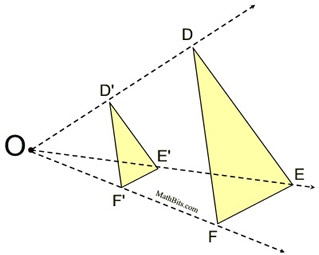The diagram at the left shows a dilation of scale factor of ½. Which segment is parallel to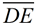?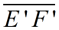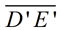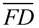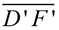9.
Which of the following statements is NOT true?

 Dilations create similar figures. Dilations preserve angle measure. Dilations may have a negative scale factor. Dilations on a coordinate axis are always centered at the origin.

10.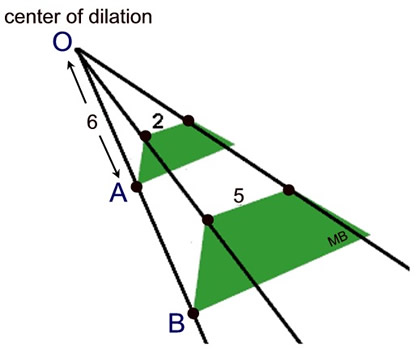Under the dilation shown at the left, what is the value of AB ?

 6 9 12 15